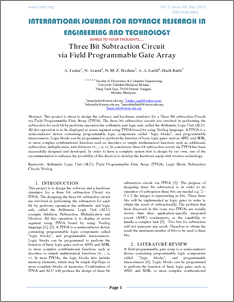Browse By Repository: Browse by Year Browse by Subject Browse by Division Browse by Author Browse by Type Statistic

# THREE BIT SUBTRACTION CIRCUIT VIA FIELD PROGRAMMABLE

Jaafar, Anuar and ARASID, NOORAISYAH and Hashim, Nik Mohd Zarifie and Abdul Latiff, anas and Abdul Rahim, Hazli Rafis (2013) THREE BIT SUBTRACTION CIRCUIT VIA FIELD PROGRAMMABLE. THREE BIT SUBTRACTION CIRCUIT VIA FIELD PROGRAMMABLE GATE ARRAY, 1 (9). pp. 1-13. ISSN 2320-6802Preview
PDF
Three_Bit_Subtraction_Circuit.pdf - Accepted Version

## Abstract

This project is about to design the software and hardware simulator for a Three Bit subtraction Circuit via Field Programmable Gate Array (FPGA). The three bit subtraction circuits are involved in performing the subtraction for each bit by performs operation the arithmetic and logic unit, called the Arithmetic Logic Unit (ALU). All this operation is to be displayed at seven segment using FPGA board by using Verilog language. A FPGA is a semiconductor device containing programmable logic components called "logic blocks", and programmable interconnects. Logic blocks can be programmed to perform the function of basic logic gates such as AND, and XOR, or more complex combinational functions such as decoders or simple mathematical functions such as additional, subtraction, multiplication, and divisions (+, -, x, ÷). In conclusion, three bit subtraction circuit via FPGA has been successfully designed and developed. In order to have a complete system that is design by our own, one of the recommendation to enhance the possibility of this thesis is to develop the hardware equip with wireless technology.

Item Type: Article Q Science > QA Mathematics > QA75 Electronic computers. Computer science Faculty of Electronics and Computer Engineering > Department of Computer Engineering ANUAR JAAFAR 22 Apr 2014 07:53 28 May 2015 04:07 http://eprints.utem.edu.my/id/eprint/9950View Item